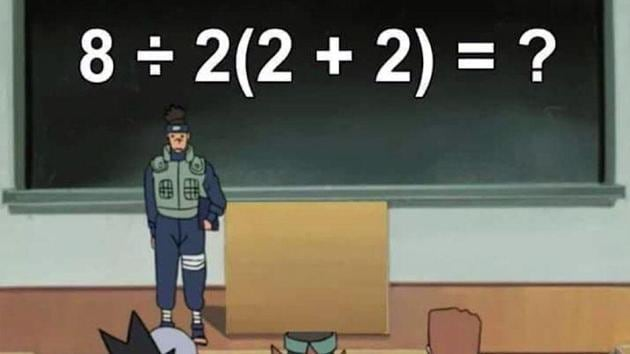The equation has started a war of sorts among netizens. Can you calculate the right answer?(Twitter/@pjmdoll)

# Simple math equation baffles people. How quickly can you solve it?

Netizens have been left scratching their heads for the correct answer to this simple question and chances are it’ll leave you confused too.
Hindustan Times, New Delhi | By HT Correspondent
UPDATED ON AUG 01, 2019 12:06 PM IST

Mathematics has always had its fair share of haters, probably because it’s hard to solve sometimes. This equation going viral is a perfect example. Netizens have been left scratching their heads for the correct answer to this simple question and chances are it’ll leave you confused too.

The seemingly simple equation was shared on July 29 by Twitter user @pjmdoll. As soon as the equation was posted, it started a war of sorts among netizens. Several people began posting what they thought could be the right answer to the equation. Why don’t you give it a go and solve the equation below:

Did you get an answer? The equation, since being shared, has collected over 7,500 ‘likes’ and more than 1,500 retweets. Interestingly, the comments section of the post is flooded with probable answers. While some say the answer is 1, a few are saying it is 16.

A similar equation appeared few days ago on Twitter which puzzled people. The equation came with a disclaimer “solve carefully” and was posted by twitter user @kj_cheetham.

What do you think is the correct answer to the equation?

SHARE THIS ARTICLE ON
Topics
Close
TRENDING TOPICS
SHARE
Story Saved